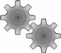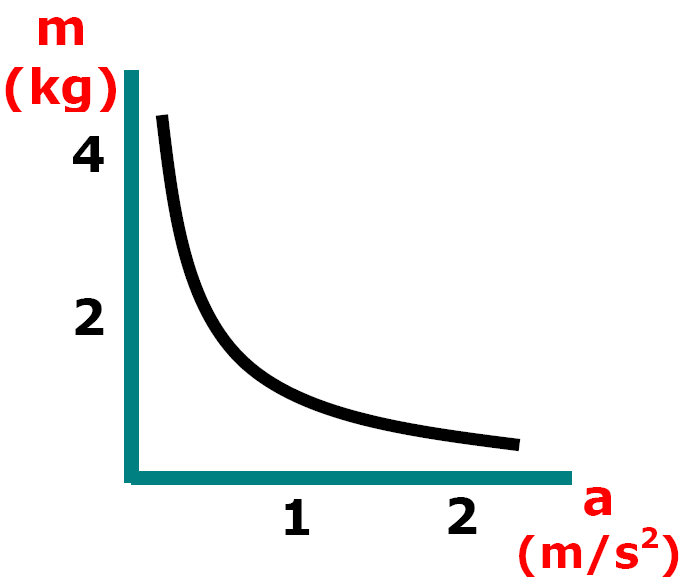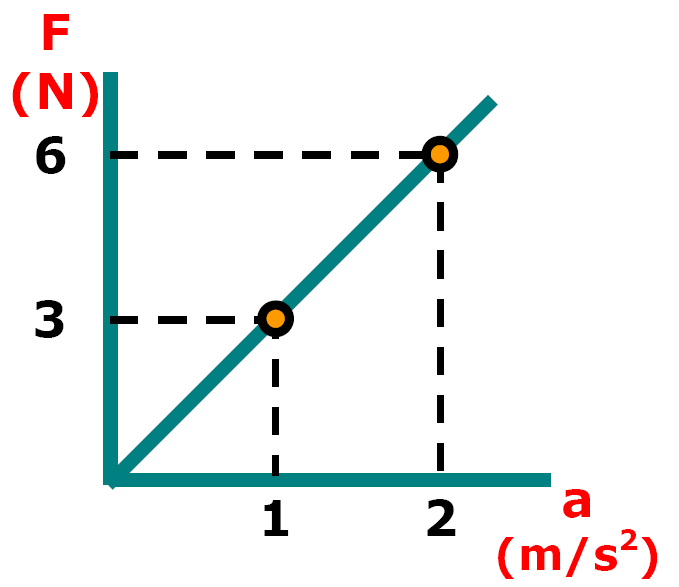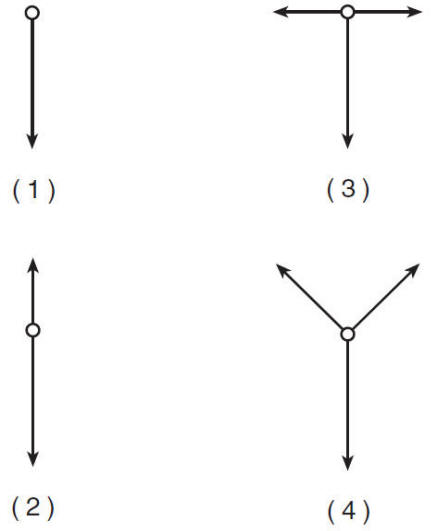HomeSimulating Zero g

What's a Vomit Comet? NASA2008 Sandia Corporation

NASA and B.Y.U.(m) a = Fnet (N) (s2) m (Kg)

2.  The acceleration a given force will produce on an object is inversely proportional to the mass of the object

[ Flash ]F = 10 nt F = 10 nt m = 2 kg m = 4 kg a = 5 m/s2 a = ? a = 5 m/s2 a = 2.5 m/s2Double the mass,
half the acceleration

 a m x2 x1/2 x1/2 x2 x1/3 x3 x3 x1/3 x1/4 x4 x3/2 x2/3"Why do all bodies accelerate at a rate of 10 m/s2 (9.8 m/s2)" (neglecting friction)Paul Hewitt3. Inertial mass (solve for m) - Finding mass using motion and force

 m = Fnet aWhat is the mass of the body
that produced the plot above?

 m = Fnet = 6 N a 2 m/s2

m = 3 kgReview RankingTask Rank the Net Force on each object from greatest to least.June 2015Force QuestionsEnrichment